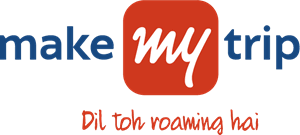Bob And Array Queries
Tag(s):

## Advanced Data Structures, Data Structures, Segment Trees

Problem
Editorial
Analytics

Bob has an array A[] of N integers. Initially, all the elements of the array are zero. Bob asks you to perform Q operations on this array.

There are three types of operations that can be performed:

1.  1 X: Update the value of A[X] to  2 * A[X] + 1.
2.  2 X: Update the value A[X] to$\lfloor$ A[x]/2 $\rfloor$, where $\lfloor$$\rfloor$ is Greatest Integer Function.
3.  3 X Y: Take all the A[i] such that  X <= i <= Y and convert them into their corresponding binary strings. Now concatenate all the binary strings and find the total no. of '1' in the resulting string.

Note: It is guaranteed that there is at least 1 type-3 query in the every test case. All the array elements will fit into 64 bit integers.

Input Format:
First line consists of two space-separated integers N and Q.
Next, Q lines consist of Q operations of either of the 3 types as described above.

Output Format:
For each type-3 query print the answer that is the no. of '1' in the resulting string.

Constraints:
$1 \le N,Q \le 500000$
$1 \le X \le Y \le N$

SAMPLE INPUT
5 5
1 1
1 2
1 3
3 1 3
3 2 4

SAMPLE OUTPUT
3
2

Explanation

Initially, A=[0,0,0,0,0]

After 1st query, A=[1,0,0,0,0]

After 2nd query, A=[1,1,0,0,0]

After 3rd query, A=[1,1,1,0,0]

For 4th query, no. of '1' in binary representation of A=A=A=1. So, answer=3.

For 5th query, answer is 2.

Time Limit: 1.0 sec(s) for each input file.
Memory Limit: 256 MB
Source Limit: 1024 KB
Marking Scheme: Marks are awarded when all the testcases pass.
Allowed Languages: Bash, C, C++, C++14, C++17, Clojure, C#, D, Erlang, F#, Go, Groovy, Haskell, Java, Java 8, Java 14, JavaScript(Rhino), JavaScript(Node.js), Julia, Kotlin, Lisp, Lisp (SBCL), Lua, Objective-C, OCaml, Octave, Pascal, Perl, PHP, Python, Python 3, Python 3.8, R(RScript), Racket, Ruby, Rust, Scala, Swift-4.1, Swift, TypeScript, Visual Basic

## CODE EDITOR

Initializing Code Editor...

## This Problem was Asked inChallenge Name

MakeMyTrip Java Developer Hiring Challenge

OTHER PROBLEMS OF THIS CHALLENGE
• Basic Programming > Bit Manipulation
• Algorithms > Searching